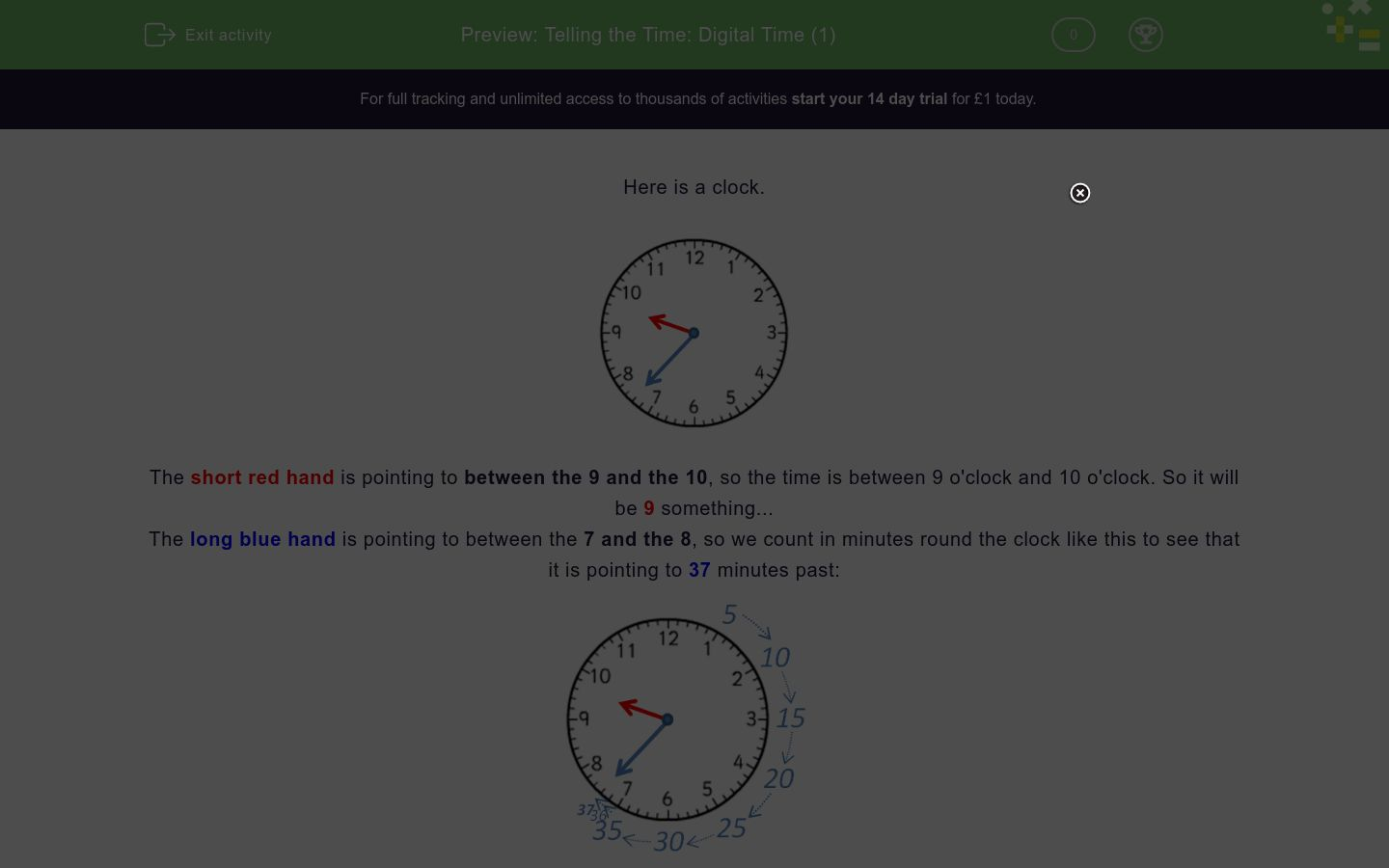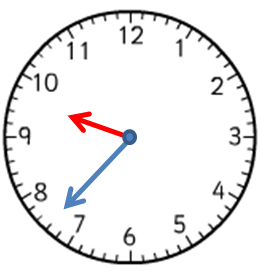# Telling the Time: Digital Time (1)

In this worksheet, students must select the digital time that matches the given clock face and the situation described.Key stage:  KS 2

Curriculum topic:   Measurement

Curriculum subtopic:   Convert Analogue/Digital Time

Difficulty level:### QUESTION 1 of 10

Here is a clock.The short red hand is pointing to between the 9 and the 10, so the time is between 9 o'clock and 10 o'clock. So it will be 9 something...

The long blue hand is pointing to between the 7 and the 8, so we count in minutes round the clock like this to see that it is pointing to 37 minutes past:The time shown is 9:37.

In the morning, this will be 9:37 a.m. or 09:37.

In the evening, this will be 9:37 p.m. or 21:37.

Remember

Add 12 to the hours for times after 12 noon in the 24-hour clock.

4 p.m. is 4 + 12 = 16:00

9 p.m. is 9 + 12 = 21:00

11 p.m. is 11 + 12 = 23:00 etc.

Want to understand this further and learn how this links to other topics in maths?
Why not watch this video?

---- OR ----